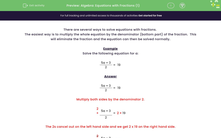# Solve Equations with Fractions

In this worksheet, students will solve equations with fractions.Key stage:  KS 3

Curriculum topic:   Algebra

Curriculum subtopic:   Understand Expressions, Equations, Inequalities, Terms and Factors

Popular topics:   Algebra worksheets

Difficulty level:#### Worksheet Overview

There are several ways to solve equations with fractions.

The easiest way is to multiply the whole equation by the denominator (bottom part) of the fraction.  This will eliminate the fraction and the equation can then be solved normally.

Example

Solve the following equation for a:

 5a + 3 = 19 2

 5a + 3 = 19 2

Multiply both sides by the denominator 2:

 2 × 5a + 3 = 2 × 19 2

The 2s cancel out on the left-hand side and we get 2 x 19 on the right-hand side:

 2 × 5a + 3 = 2 × 19 2

Simplify:

5a + 3 = 38

Subtract 3 from both sides:

5a + 3 - 3 = 38  - 3

Simplify:

5a = 35

Divide both sides by 5:

5a ÷ 5 = 35 ÷ 5

Simplify

a = 7

Let's practise this in the following questions.

### What is EdPlace?

We're your National Curriculum aligned online education content provider helping each child succeed in English, maths and science from year 1 to GCSE. With an EdPlace account you’ll be able to track and measure progress, helping each child achieve their best. We build confidence and attainment by personalising each child’s learning at a level that suits them.

Get started